An estimator for the road freight handling factor

Thomas Cool
March 3 1997

Transport Research Center
Ministry of Transport, Public Works and Water Management

P.O. Box 1031
3000 BA Rotterdam
The Netherlands

Summary

Analysis of road freight transport is hindered by the lack of direct observations of the handling factor. The handling factor is the frequency of lifts of the tonnes in the supply chain from origin to destination. It also is the number of times that the same tonnes are reported to the central statistical office. Changes in the tonnes lifted figure may be caused by changes in the actual tonnes in transport or by changes in the handling factor, in unknown proportions. An econometric technique can be used to estimate what has not been observed, and thus to recover the hidden variables of both the handling factor and the tonnes in transport.

Thanking Henri Dijkman, Hans Flikkema, Bob Knippenberg, Hans van der Rest and two anonymous referees for their valuable comments.

Contents

An estimator for the road freight handling factor

Summary

Contents

1. Introduction

2. Concepts

3. Model

4. Estimation result

5. Graphs of the result

6. Extrapolation

7. International transport

8. Perspectives on further research

8.1 Rates of change and cointegration

8.2 Sectoral analysis

8.3 Other factors

8.4 The level

9. Conclusion

Literature

Data appendix

1. General input

2. Inland transport 1963-1993

3. International transport 1979-1993

1. Introduction

The analysis of road freight transport focusses on the tonne-kilometers. The tonne-kilometers themselves are explained by the tonnes lifted and the average distance per lift. And the tonne-kilometers together with the average load per lift explain the vehicle kilometers. So, with the tonne-kilometers at the core of analysis, there is great interest in finding reliable forecasts of it. In principle one would like such forecasts to fit in with the structural determinants of the variable. However, it turns out that tonnes lifted and average distance are rather unreliable variables. They are influenced in uncertain ways by the handling factor. In other words, analysis of road freight transport is hindered by lack of reliable data on the handling factor.

The handling factor is the frequency of lifts of the tonnes in the supply chain. It hence is the frequency by which the same goods are handled from origin to destination, and thus it is, if statistical measurement is correct, also the number of times that the same tonnes are reported to the statistical office. Algebraically, the handling factor is the ratio of the tonnes lifted to the actual tonnes passing through the supply chain. For clarity of terms, the latter tonnes in the supply chain will be called the tonnes in transport.

It can be deduced - as will be done below - that the handling factor also is the ratio of the integral distance over all the lifts to the average distance of each lift. The integral distance is the distance from producer to the final customer. Note that the integral distance is an average in itself too, i.e. an average of all traffic. But instead of saying "average integral distance" it suffices here to say "integral distance".

The statistical observations made by the Dutch Central Statistical Office (CBS) on inland transport concern the tonnes lifted and the tonne-kilometers. These data do not provide a full summary of developments in transport, and neither is it possible to derive the handling factor by applying a fixed accounting rule or formula to the two kinds of data that are given. Also, the data on tonnes lifted and tonne-kilometers are affected in uncertain ways by changes in distribution (here: production and logistics), and by influences of these changes on the reports made by the economic agents to the Central Statistical Office. For example, if the number of distributors doubles and the average transport distance halves, and all else such as the integral distance remains the same, then these distributors would report twice as many tonnes lifted, though the number of tonnes in transport would not actually change. Another example is that when the CSO / CBS reports a rise in the tonnes lifted, one time it might mean a change in the tonnes in transport, and another time it might mean a change in the handling factor. Since the handling factor is unknown, uncertainty abounds, not only in the size but also in the kind of development. Hence the statistical summary would be more complete if there would be information on the handling factor.

One approach to tackle this data problem is the use of econometrics, and notably to devise structural equations with hidden variables and then to estimate what has not been measured statistically.

One of the main assumptions below will be to relate the tonnes in transport to the national product. Another assumption is that the integral distance will depend upon income and on developments within the distribution system.

It may be noted here that the present analysis concerns unimodal transport of road freight only. Thus we do not regard multimodal chain transport where the integral distance from origin to destination has a common meaning, as in Chen & Lenoir (1995). However, in our analysis, origin and destination are defined by the statistical procedures of the system of national accounts, and thus have a clear meaning. In our analysis the "final customer" need not be a consumer, but will be the "customer implied by the data generating process".

Section two clarifies the concepts used. Section three develops the structural model. The simultaneous estimation on aggregate data for Holland for 1963-1993 appears to give interesting estimates, as reported in section four. To judge on the matter, section five plots some graphs of the results, and section six gives a trend extrapolation.

Section seven contains an estimate of elasticities of Dutch international transport for the 1979-1993 period. The handling factor of international transport can be taken as 1, reflecting the fact that cross-border transports are reported only when the goods cross the border. There may be many hauls before and after the goods cross the border, but the statistical procedure only records the haul precisely across the border. The income and distance elasticities of international transport provide an indication for the inland transport that we are concerned with here.

Section eight comments on the possibilities for further research.

Some information appears to support the estimation results indeed. However, we should use data on the meso and micro level, also on other modes of transport, and on prices and costs. So we can only conclude, in section nine, to cautious confidence.

2. Concepts

The subject of the handling factor concerns concepts that are rather alike, but not precisely so. Imprecision in this subject gives a greater chance of confusion than is the case in other subjects. This section may help to prevent such confusion. Even though the examples given below are likely too simple to keep one’s attention and even may be insulting to academic intelligence, it may still be advisable to digest them.

Let us regard a chain from factory, wholesaler, local warehouses and shops, and this over a period of two years. Let the factory produce 10 tonnes, and let these be transported over a distance of 90 kilometers to the shops. The wholesaler is 50 kilometers from the producer and 40 kilometers from the shops. In year 1 there are various local warehouses before the goods actually reach the shop. In year 2, the warehouses are skipped, and the goods are transported ‘just in time’ to the shops directly from the wholesaler. Given that there are fewer hauls from factory to shop, there is a concentration in terms of the handling factor. This example has been chosen such that, for convenience, the integral distance from the factory to the shops does not change and remains 90 kilometers in both years. Table 1 contains the data of this example. The table contains a "total" that needs some discussion below.

Table 1: Example of concentration in terms of the handling factor

 Chain Year 1 Year 2 tonnes km tonne-km tonnes km tonne-km Producer - wholesale 10 50 500 10 50 500 Wholesale - warehouses 10 30 300 Warehouses - shops 10 10 100 Wholesale - shops 10 40 400 Total 30 90 900 20 90 900

Though Table 1 gives the actual data on the example problem, it appears that these data are difficult to measure. To understand the difficulty in measurement, we best regard the statistical practices by which the transporting agents are obliged to report their activities to the statistical office. They will report the tonnes lifted and the average distance per haul, i.e. the first two columns of a year in Table 1. The statistical office will determine the tonne-kilometers in the third column. Then it computes the total values of column 1 and column 3 of a year, and reports only these totals. From these, third parties can compute the average distance. The statistical report on the case of Table 1 is given in Table 2.

Table 2: Example of statistical reporting

 Year 1 Year 2 tonnes km tonne-km tonnes km tonne-km Total 30 30 900 20 45 900

We find two crucial points when comparing Table 1 and Table 2. The first is that Table 1 gives the integral distance (90 km) while Table 2 gives the average distance (30 or 45 km, depending upon the year). The second is that the totals row in Table 1 allows the determination of the tonnes in transport (10 = 900 / 90), while the totals row in Table 2 doesn’t. These two crucial points are related. Given Table 2, the tonnes in transport can be determined from the integral distance, and vice versa.

Given the subject of this paper we ask: What is the handling factor in this example ? The handling factor is given by the frequency of lifting the tonnes in transport. Hence in year 1 it is the ratio 30 / 10 = 3, and in year 2 it is 20 / 10 = 2. Thus the statistical office reports a drop in tonnes lifted, while, paradoxically, the actual tonnes in transport have not changed. The paradox can be explained: changes in the amount of tonnes lifted have ambiguous meaning, and the handling factor is needed to supplement the picture.

Note that it suffices to know only one of these three - the handling factor, the integral distance and the tonnes in transport - to be able to determine the other two.

Above example is quite simple. It regards one flow of commodities, while transporting agents normally transport more goods in more directions (with more modes of transport). Obviously it would make for a very complex task to keep track of many movements in all kinds of directions. Precisely since that would be a very complex task, the statistical practice has been simplified to the format of Table 2. The most important variable, the tonne-kilometers, has been recorded. This simplification (however) comes at a cost, namely the lack of information on the handling factor, the integral distance and the actual tonnes in transport in the supply chain.

In contrast to above simplicity, reality is complex. It is useful to emphasise the role of complexity. Figure 1 contains a diagram of only 5 nodes, labeled A till E. The arrows between the nodes only give the direct hauls. Longer hauls, e.g. from A to B to D, are not depicted. Most longer hauls are likely to be regarded as detours, but they still might be efficient in terms of carrying capacity.

Figure 1: Only five nodes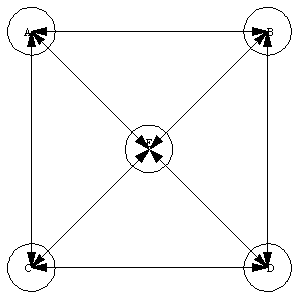We might assume that A and C are sea coast villages and that B and D are inland patato growing settlements. There will be trade between A and C on one side and B and D on the other side, to provide the optimum levels of fish and chips in all places. When transport costs are high, A will only trade with B, and C will only trade with D. Due to differences in population sizes, technologies and tastes, the patato/fish relative price p(A, B) will differ from p(C, D). When transport costs drop, flows will start in various directions and there will be an equalisation of these prices. Major flows along the A - B and C - D branches are likely to remain intact, and it is also likely that E develops into a market place at least for surplus or speculation amounts. Events however are not unambiguous. As said, long hauls are possible, such as trips around the square in both directions. Finally, it is interesting to wonder what happens when the external nodes start specialising. Fish and patatoes can be specialised for consumption and for production purposes, and in that case E is likely to grow more important as a distribution center.

(Note: Krugman (1996) gives an example of a simulation technique to tackle complexity directly. Here, however, complexity is only mentioned to better understand the notion of the handling factor.)

The handling factor summarises a simple aspect of a complex system, namely the average number of times that tonnes are lifted from origin to destination. Its simplicity is both its strength and its weakness. The following points may be noted.

First of all there are also other measures of complexity. For example one measure is the total number of agents active in distribution. There is no direct relationship between the handling factor and the number of distributors, or actors in the chain of supply. A change of the number of distributors need not affect the handling factor, at least when the kind of chain and the number of hauls remain the same. A change of the handling factor need not affect the number of distributors, when they only change their role. For example in Table 1, the change from year 1 to year 2 may have occurred since the warehouses have been relocated and have changed their official name from warehouse into wholesaler, but not their number of small enterprises or employees. So, when discussing ‘complexity’, it is necessary to be clear on what measure of complexity. When the handling factor is measure of complexity, then it is tautologous that a higher handling factor is related to higher complexity (in terms of the handling factor itself). However, one can imagine cases where complexity increases (in some other definition) and the handling factor drops.

Secondly, a change of the handling factor does not clarify in itself what that change means at the micro level, and why it occurs. A drop in the handling factor does not tell us whether it happens in the North or in the South, upstream or downstream. It neither gives the cause. It is a summary statistic.

Thirdly, there is the interaction of changes in distribution (production and logistics) and changes in statistical observation. When products grow more sophisticated they tend to require more stages in distribution. In statistical observation, these stages may be reclassified to other sectors of production, and thereby there may be less insight in the actual stages.

The latter point is sufficiently important to discuss it at some greater length. Let us also use an example to discuss it. Regard Figure 1 for two years, and record the following situations in Table 3. In year 1 raw fish is shipped from A to E and then from E to D. Thus there are two hauls and a handling factor of 2. In year 2 raw fish is shipped from A to E. There it is processed, put on the market and then shipped from E to D under the statistical label of "processed food". Thus there are two hauls again, but it becomes a question what the handling factor would be or should be. A straightforward approach - called ‘the chain view’ - will be to say that it is 2 again. The idea is that the tonnes are transported subsequently, so that they are part of a chain. Alternatively, there is the approach - called ‘the product view’ - that raw fish and processed food are different, so that the "tonnes in transport" must be measured as 10 + 10 = 20 tonnes. In the product view the handling factor in this example is 1 instead of 2.

Table 3: Example of sophistication

 Chain Year 1 Year 2 tonnes km tonne-km tonnes km tonne-km raw fish A - E 10 1 10 10 1 10 raw fish E - D 10 1 10 processed food E - D 10 1 10 Total 20 2 20 20 2 20

Sophistication in distribution thus causes two points of view, the chain and the product point of view. Discussion of and between these views is highly academic, since there are few actual observations of the tonnes in transport - and what has been observed already presupposes a point of view and thus cannot be used as evidence. Much is a matter of definition. And having taken a definition, the main thing is to remain consistent.

It must be noted, though, that the product and chain views both have extreme positions that put a burden on consistency. In the product view, a simple relabeling of a product (putting on another brand sticker) already causes a new product, and thus an addition to the ‘tonnes in transport’. In the limit, the product view becomes meaningless, since each haul means a change in the location, and - since location is an important aspect of an economic commodity - essentially a new product. In the limit then there is no distinction between the tonnes lifted and the tonnes in transport. On the other hand the chain view also has an extremist position. This is, that one must take account of leaks out of and into the chain. If some inputs or outputs of products are not measured properly, e.g. input of rainwater and output of smoke, then the chain gets distorted. In the limit the product view becomes meaningless, since there is no final destination. These extremist positions however do not hinder practical research. Practical work uses classifications of products, so that some sophistication is allowed for, but up to a level. The condition of consistency then means that one must recognise the limitations of the statistical procedures. Once a system of observation is in place, the tonnes in transport and handling factor are well defined in terms of that system, and can be used consistently. The practical problem becomes, in this very moment, to find reliable forecasts on commonly used statistical measures on tonnes lifted and tonne-kilometers, and notably the latter.

Below we will look at road freight in Holland and use statistical observations on the tonnes lifted and tonne-kilometers. We will develop an estimator of "the" handling factor. This estimator is based on the assumption that the tonnes in transport tend to grow with the national product (measured by the commonly used economic indicator). This handling factor is not extremist - it adheres to neither the chain nor the product point of view - but it is oriented to practical use. It is difficult to pinpoint its position in the spectrum created by the extremist views. In a sense it is just ‘the handling factor that follows from the formulas and estimation routine’. Its value as a tool then depends both upon the forecasting qualities and upon the degree of belief that one can put on the statement that the tonnes in transport are associated with national income. However, given that national income is the major variable considered in the practice of forecasting transport, the use of the "the" label might be warranted. Thus: an estimator for the handling factor; in short for: an estimator for a national income based handling factor.

The estimator presented here is related to the value added density in transport. The value added density is the ratio of value added to the tonnes in transport. In discourse about the ‘dematerialisation of society’ it is often remarked that national product (measured in value added) increasingly originates from services. Material production, associated with the tonnes in transport, would lag behind. In that case the value added density would show a rising trend - and rising with national income itself. It is this trend that can be used to arrive at an estimator for the handling factor.

3. Model

The central statistical office CBS (1987 etc.) observes the tonnes lifted (TL) and the associated tonne-kilometers (TK), so that we can deduce the average transport distance (A):

TK = A . TL (1)

Figure 2 plots the development of the observed variables as indices with 1963 = 1. Though there will be many causes affecting this development, we will concentrate on gross national product, and it therefor is included in the graph. In Figure 2 the tonnes lifted are below and the tonne-kilometers above national income.

Figure 2: Development of tonnes lifted (below) and tonne-kilometers (above),
both of inland transport, and national product (middle), Holland 1963 = 1Source: CBS, see data appendix

Figure 3 gives the same data as Figure 2 but then for 1979 = 1. Figure 3 better shows that Dutch national income and transport slowed in the wake of the oil crises in 1973 and 1979. The development in 1974-1984 is rather flat. However, the overall growth of the tonne-kilometers is much stronger than the growth of national income and tonnes lifted.

Figure 3: Development of tonnes lifted and tonne-kilometers
of inland transport, and national product, Holland 1979 = 1Source: CBS, see data appendix

One may note the divergence of TK and TL - which divergence means a rise of the average transport distance. It is interesting to develop a plot for the average transport distance. Note that (1) can be transformed as follows:

Log(TK) = Log(A) + Log(TL) (1’)

so that a plot in the (Log(TL), Log(A)) space has the easy property that adding the values of the co-ordinates generates the Log(TK) value. Thus, the contour lines are negative diagonals. Figure 4 contains that plot, with the annual movement indicated by arrows, and a dashed example contour. The graph indicates some larger jumps in the 1960s and in 1988.

Figure 4: Development of tonnes lifted and transport distanceSource: CBS, see data appendix

Figures 2 till 4 show some large fluctuations in the actual observations made by the Dutch statistical office CBS. It would be interesting to relate these to possible changes for example in regulations, size of vehicle, modal competition and economic structure. All these have an impact on the handling factor. However, the objective of this paper is to develop the estimator for the handling factor that links primairily to the national product.

Unobserved are the tonnes in transport (T), the handling factor (h) and the integral distance (K):

h = TL / T (2)

K = TK / T (3)

From above it follows:

K = h .A (4)

The transport distance (A) depends negatively on the handling factor in two ways. Partly it is a matter of definition. When the integral distance (K) and the tonnes in transport (T) remain the same (‘all else equal’), and the handling factor (h) doubles, then the tonnes lifted (TL) double and the statistical average of the distance (A) is halved. Apart from this definitory relationship there will be a behavioural component too. Parameters will determine the deviation from the definitory relationship. These parameters control the growth of tonne-kilometers as a result of changes in logistics.

A = h . e - q h (5)

Another behavioural assumption is that the number of tonnes in transport grows with both national income (y) and a trend for the value added density - i.e. value added per tonne (y / T):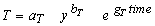(6)

The integral distance will change with those same variables and the handling factor: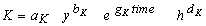(7)

These assumptions derive from demand theory and, for h, on the assumption of a lognormal distribution - related to ‘power law’ regularities. There is no prior information on the sign of dK.

Combining these definitions and behavioural assumptions results into the following two equations for simultaneous estimation. Firstly (3) can be written as TK = T.K, and substitution of (6), (7) and then (5) gives, changing to logarithms:

Log(TK) = Log(aT + aK ) + (bT + bK ) Log( y) + (gT + gK ) time +

+ dK Log( Log(h / A) / q ) (3’)

The last term in (3’) can be simplified, but has been left in this form so that one can check more easily that it is the inverse of (5). Secondly, taking (5) and (6), changing to logarithms:

Log(A) = Log(h ) - q TL /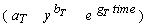(5’)

The crucial insight is this. By proper substitutions we have eliminated the unknown handling factor h. We have produced two equations in known variables that allow the identification of key parameters. The estimated values of these parameters then can be applied to the right hand side of (6) to generate an estimate of the tonnes in transport. Since it suffices to know only one of the three unknown variables, we then can easily derive the remaining unknowns, i.e. the handling factor via (2) and integral distance via (3) or (4) or (7).

It may be observed that other kinds of substitutions and combinations can generate other equations for estimation. Above selection seems most robust - while it is useful to have a simultaneous test.

Finally, one can check that all parameters are identified up to aT. That means that the level of the handling factor or the level of the integral distance cannot be determined by above relationships.

An estimator of the level of the handling factor however is useful. The least that we can do is normalisation. Simple dispersion cannot be used, since when a variate x is known up to a scalar, also its average and standard deviation are unknown up to that same scalar. An estimate however can be found by assuming maximum entropy. Since h is equal or greater than unity (by definition), then f = 1 / h is a fraction equal or less than unity, and the entropy measure is (assuming that it is relevant for h only):

Entropy = - f . Log(f) = Log(h) / h (8)

Entropy reflects uncertainty. Choosing a maximum entropy normalisation here is another way of saying that we present the estimator that best reflects our actual lack of knowledge of the true level of the handling factor.

4. Estimation result

Equations (3’), (5’) and (8) have been estimated for the 1963-1993 period. The correlation coefficient is 99.998%, but this value has limited meaning due to the use of levels instead of growth rates. In fact, due to the estimated constants we will see high correlation throughout.

Two runs appear to be necessary. In the first run aT is not known, and auxiliary variables must be used for combinations of parameters. In the second run the level of aT can be plugged in. The t-values slightly change due to the larger Hessian matrix. Table 4 reviews the results.

The t-values are more important than the correlation, and these are encouraging. The coefficients can then be evaluated as follows:

• The tonnes in transport T have an elasticity bT = 1.12 on national income and a trend gT = .65%. These values are reasonable, with an appealing ratio.
• The integral distance K has a zero elasticity on national income; i.e. the value bK = .033 is statistically insignificant. But there is a trend gK = -1.4% and an elasticity on the handling factor dK = -.39, thus an effect close to 1 / Ö h.
These latter negatives are a bit surprising. But it may be remarked that the definitory relationship implies an elasticity of -1, so that the value of -.39 implies a ‘real’ contribution of .61 (so that a rise of h ‘partially’ raises K).
• The transport distance A has an elasticity on the handling factor of -q = -.27 times that handling factor. The ‘real’ contribution thus is a proper positive .73.

Table 4: Result of simultaneous estimation in two runs

 Coefficient value t-value run 1 t-value run 2 constant 9.12109 134.654 - q / aT 0.00467093 8.09835 - Log(h ) 4.67595 31.5156 - aT 59.6097 - entropy bT 1.1207 11.0865 9.08799 gT 0.00655008 1.32689 0.905735 aK 254.395 - 3.6672 bK 0.0336525 0.238815 0.153789 gK -0.0145471 -2.43263 -1.2169 dK -0.395451 -2.45761 -2.06577 h 107.335 - 4.26507 q 0.278433 - 5.15231

5. Graphs of the result

Figure 5 shows the estimated handling factor, reflecting an apparent concentration. Note that the level is less certain than the development.

Figure 5: The handling factor drops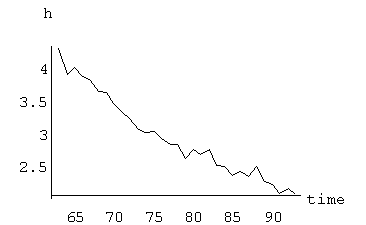Source: computed from the estimated parameters

The NEA (1991) study gives a handling factor of 2.54 for 1983 (only), though for all freight (e.g. including ships). This corroborates above result, since road freight has a large share.

Figure 6 combines various results. The dashed line is the definitory relationship, when the tonnes in transport are constant and the measurement of tonnes lifted would double when the handling factor doubles. The smooth curve is the estimated equation (5) which explains the development of the average transport distance. Finally, the plot with the wilder fluctuations is the result of combining Figure 5 with the actual observations on A. Note that 1963 is in the lower right corner and 1993 in the upper left corner, reflecting the concentration of trade and production.

Figure 6: Average transport distance as function of the handling factor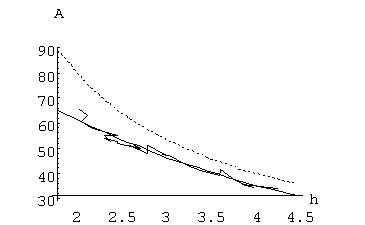Source: computed from the estimated parameters

Both equation (6) and (3) can be used to compute T. This result is plotted in Figure 7. The error between both approaches seems negligible. With respect to Figure 2 one can observe that T closely follows TK, since, apparently, K is relatively stable.

Figure 7: Two measures for the tonnes in transportSource: computed from the estimated parameters

Similarly, both equation (7) and (4) can be used to compute K. This result is plotted in Figure 8 (with (7) dashed). The error between the two estimates is maximally about 15 kilometers (Holland is a small country). Figure 8 suggest that the integral distance has shrunk from 140 to 130 kilometers. Of course, the development is more certain than the level.

Figure 8: Two measures for the average integral distanceSource: computed from the estimated parameters

Figure 9 gives the Log-Log plot for T and K. Due to the plotting range, the dashed example contour line doesn’t look like a negative diagonal, though it is one. The impression is that K is rather stable till 1974 (first oil crisis) and then starts dropping - though slightly and gradually.

Figure 9: Tonnes in transport and integral distanceSource: computed from the estimated parameters

6. Extrapolation

Our analysis of the handling factor can proceed one step further. Substitution of the parameters gives us an estimate h = TL / T(y) as plotted in Figure 5. We like to smooth this estimate, and extrapolate it. It is reasonable to assume an exponential decrease with the logical limit value of 1. This leads to the following assumption: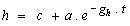(9)

Table 5 gives the estimation results with a correlation coefficient of again 99%.

Table 5: Trend estimate of the handling factor

 Coefficient value t-value c (limit) 1.40703 6.90705 a 2.90244 17.0189 gh (trend effect) 0.0468933 7.22835

Plotting this, with an extrapolation for the next 20 years, gives Figure 10. Note that assuming constancy of the factor for the next 20 years causes an error of only 8% in K. Of course, equation (9) relates the handling factor to a trend term only, which is not causal. One can imagine economic developments that actually increase the handling factor. It seems, though, that these have not materialised yet in any great strength.

Figure 10: Trend estimate and extrapolation of the handling factor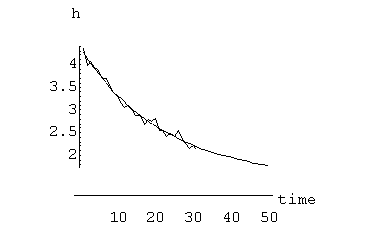Source: estimated on estimated variable

7. International transport

As mentioned in the introduction, it can be assumed that international transport has a handling factor equal to 1, since the tonnes are only measured when they actually cross the border. It is useful to present some estimates - while assuming this unit handling factor - since the international elasticities help judgement on inland transport. Unfortunately, consistent data exist only for the 1979-1993 period. These data are plotted in Figure 11.

Figure 11: Development of tonnes lifted (middle) and tonne-kilometers (above),
both of international transport, and national product (below), Holland 1979 = 1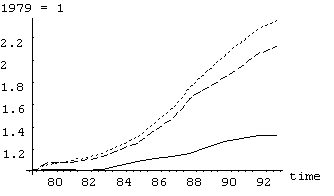Table 6 contains the estimation results. The elasticities are with respect to national income and not exports and imports. These results come from estimating the levels and not rates of change, and the reason for selecting this method is that these results seem more reliable.

Table 6: Elasticities for international transport 1979-1993

 macro value t-value bT 1.48306 6.26892 bK 0.150865 0.637709 gT 0.0230811 4.05059 gK 0.00538407 0.944872

International road freight is more sensitive to national income than inland road freight, both in tonnes and in distance (while K = A). There also are important positive trends in that period. These values seem to capture common notions, and also relate usefully to the inland estimates. (The conclusions section below contains a table with both results, for comparison.)

Figure 12 contains a plot of the (Log(TL), Log(A)) = (Log(T), Log(K)) space. The observed path shows some fluctuations, while the estimate generates an almost straight line.

Figure 12: Development of international tonnes lifted and transport distance,
observed and estimated, 1979-1993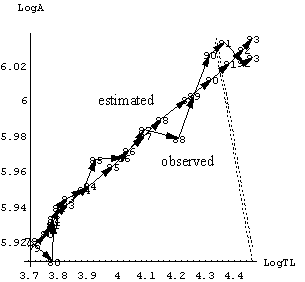8. Perspectives on further research

8.1 Rates of change and cointegration

The equations in the model can be manipulated in more ways. Above estimation has been done in levels, but usually we are interested in estimation by rates of change too. Properly, the model should be estimated using cointegration techniques that allow for the influence of both levels and rates of change.

Let us first look into a rates of change specification.

Let us take (3) TK = T.K, substitute (7) for K, eliminate h = TL / T, and then substitute (6) for T. Taking rates of change then gives an expression that is related to (5’):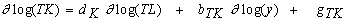(10)

with e.g.: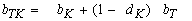(11)

Note that one of the advantages of the present analysis is that we now have an interpretation of the Log estimation result of (10), namely an interpretation in terms of relations (6) and (7).

Estimating (10) gives a correlation coefficient of 72.1%. Given this estimate, a coefficient of the level can be estimated, with a correlation of 99.99%. Finally, equation (5’) can be estimated separately, with a correlation of 96.5%. Collecting these partial results gives the total result of Table 7.

Table 7: Stepwise, using rates of change

 Coefficient value t-value type of estimate constant 6.55527 949.564 separate, run 2 bTK 0.616657 2.4108 dlogs, run 1 gTK 0.0119317 1.23522 dlogs, run 1 q / aT 0.00454049 6.98467 separate, run 3 Log(h ) 4.64219 27.6436 separate, run 3 aT 58.1004 - entropy bT 1.12418 9.66764 separate, run 3 gT 0.00745787 1.23244 separate, run 3 aK 75.1004 - computed bK -0.001393 - computed gK 0.00783 - computed dK 0.450221 3.02212 dlogs, run 1 h 103.771 - computed q 0.26531 - computed

Some elasticities do not differ much from the simultaneous level estimate, but dK notably has a reversed sign. (Note that there is no theory about this sign.) Though we are advised to look into the specification too, a main conclusion seems that the partial approach is less reliable.

At the moment of completing this paper, some work has been done on applying cointegration techniques. What appears to be difficult - yet - is to find proper interpretations of coefficient results in terms of the (a) structural model.

8.2 Sectoral analysis

One way to test our approach is to apply it to sectors in the economy. Perhaps the handling factor of agricultural products differs from the handling factor of chemicals, while both still satisfy the general formulas above - though with different parameters.

Our test encounters problems of model choice and data collection.

The first problem concerns the proper choice of model to allow for sectoral effects. We adapt formulas (6) and (7) by inserting an additional indicator for the gross value added of the sector itself. For example for sector i we get:(6’)

The choice of this model appears to be counter-intuitive to some, who tend to leave out the macro variable and who thus only regard the effect of production of the sector itself. However, the tonnes in transport are primairly dependent upon the demanding sectors, for which the gross national product is a proxy. We add the supplying sector only to allow for intra-industry specialisation effects. Note that the sum of the b’s will give the total income elasticity.

The second problem is that data on TK and TL are observed in NSTR commodity classification, while the data on sectoral production concern sectors of the economy as defined by the national input-output tables. The allocation of NSTR groups to sectors should be done at least at the 2 digit level. Presently only a rough 1 digit allocation has been feasible, and tried indeed. The estimation results on this are encouraging in themselves, but will not be presented here, since only a 2 digit allocation defines a proper data set.

It may be noted that the sectoral data appear to be limited to the 1979-1993 period. This is too short for cointegration techniques. So one could imagine a system where sectors are estimated by above model for the shorter run, while the macro total is determined and corrected by another model estimated by cointegration for the longer run. Alternatively, since the original reports by distributors in the earlier period are collecting dust in the archives of the CBS, it might be an idea to still compile the earlier data at the 2 digit level.

8.3 Other factors

In various places in the body of the paper we have mentioned the drawbacks of the present approach. It may be useful to collect these. Drawbacks are:

• explained are only tonnes lifted and tonne-kilometers, not vehicle-kilometers etc.
• unimodality
• only the influence of national income and a trend term
• no interaction between national and international transport
• no development of statistical measurement problems
• choice of period (test on trend changes)

Other variables to consider are regulation, size of vehicle, economic changes, prices and costs. The model is very simple on logistics. Notably, it is assumed that the handling factor has an influence on the average distance, but no factors have been included to explain the development of the handling factor itself. One could for example conceive an indicator of the sophistication of products, and relate this to the factor.

The sophistication of products would seem to increase the handling factor. But statistical reclassifications might again reduce it. It is not possible to disentangle these influences by the method adopted here.

8.4 The level

Above we have used an entropy measure to estimate the level. This mainly is an expression of uncertainty. When other factors are included, we should be able to be more specific about the level of the handling factor.

9. Conclusion

The findings can be summarised as follows. In Holland from 1963 till 1993 there seems to have been a concentration of trade and production. The average transport distance has been observed to rise from 34 to 66 kilometers. Had the system of distribution remained the same, with a handling factor of 4, then the integral distance would have risen from 140 km to 265 km. But since the system of distribution changed, and the handling factor dropped to 2, the integral distance actually shrunk, to something like 130 km. Thus there has been a concentration in logistics without an increase of the integral distance.

The latter finding is in line with the one by McKinnon & Woodburn (1995): "Approximately half the firms that had centered their operations stated that this had increased their road freight requirements; 23% believed that centralisation had not affected these requirements, while, for 28%, it had reduced them. These results cast doubt on the conventional view that the process of centralisation invariably generates additional freight transport (...)" For Holland, a KPMG (1993) study for the AVV notes that freight traffic that is generated by Just in Time practices may be countered by efficiency and specialisation: "In recent years these counter-developments have compensated for each other" (with, though, an influence of capacity utilisation).

The correlation and the t-values of the estimate are high. At this moment the results do not seem unconvincing. The result on the handling factor finds some support in the NEA (1991) study and from estimates on international trade. Table 8 summarises the estimates. It must be remarked, again, that the actual level of the (inland) handling factor recovered here is less certain than the development.

Table 8: Elasticities of road freight transport on national income

 macro bK bT bTK gK gT gTK inland 1963-1993 0.03 1.12 1.15 -1.45% 0.65% -0.80% international 1979-1993 0.15 1.48 1.63 0.50% 2.30% 2.80%

This result needs to be corroborated by more information about the meso and micro level. Also, above model is very simple on logistics. We also need to take into account other modes of transport, and the influence of prices and costs.

Hence, above result can be used with some confidence, but not without caution and not without continued research.

Literature

Centraal Bureau voor de Statistiek (1987), "Statistiek van het binnenlands goederenvervoer 1986; deel 2 wegvervoer" (Statistics of inland freight transport 1986, part 2 road transport)

Centraal Bureau voor de Statistiek (1988), "Statistiek van de aan-, af- en doorvoer 1986; goederenvervoer van en naar Nederland" (Statistics of imports and exports 1986; material transports from and to The Netherlands)

Centraal Bureau voor de Statistiek (1990), "Het bezit en gebruik van bedrijfsvoertuigen 1989" (Possession and use of company vehicles)

Centraal Bureau voor de Statistiek (1995), "Nationale rekeningen 1994" (National accounts 1994)

Centraal Planbureau (1981), "Centraal Economisch Plan 1981", The Hague

Chen, T.M. & R. Lenoir (1995), "Transportketens in goederenvervoermodellen" (Transport chains in freight models", AVV, Kenniscentrum Logistiek publicatie nr. 9

Cool, T. (1995), "Economics programs written in Mathematica", Scheveningen; ewp-prog/9508001; see: http://www.can.nl/~cool

Cool, T. (1996), "Estimating tonne-kilometers using hidden variables", Mathematica notebook, AVV

Kapur, J.N. and H.K. Kesavan (1992), "Entropy maximization, Principles with applications", AP

KPMG (1993), "Logistieke concepten van de toekomst" (Logistics concepts of the future), report in consignment of the AVV

Krugman, P. (1996), "The self-organizing economy", Blackwell

McKinnon, A.C. & A. Woodburn (1995), "Logistical restructuring and road freight growth: An empirical assessment", Transportation 22: 1-21

NEA (1991), "TEM 2, Deelrapport 2, Transformatiemodule", Rijswijk, 910090/12572

Ortuzar, J. de D., & L.H. Willumsen (1994), "Modelling transport", John Wiley, 2nd ed

Data appendix

1. General input

Dutch national income is given in the National Accounts, CBS (1995) etc. Note that we use value added at factor costs here while gross production might be better. The index 1964 = 1 is used in both estimations.

y(1964=1) = {0.923264, 1, 1.05133, 1.07795, 1.1372, 1.20557,

1.28466, 1.36718, 1.42414, 1.47509, 1.56453,

1.62134, 1.59008, 1.67944, 1.71975, 1.75572,

1.80289, 1.83013, 1.82723, 1.80331, 1.83664,

1.90198, 1.97034, 2.01475, 2.05541, 2.10611,
2.20066, 2.28923, 2.34726, 2.38855, 2.39754}

Below, TK is given in million tonne-kilometers, TL in million tonnes, and A in kilometers.

2. Inland transport 1963-1993

Dutch inland transport is given by CBS (1987) etc.

Input:

Trend = {1, 2, 3, 4, 5, 6, 7, 8, 9, 10, 11, 12, 13, 14, 15,
16, 17, 18, 19, 20, 21, 22, 23, 24, 25, 26, 27, 28, 29, 30, 31}

TL = {233.1, 233.131, 255.902, 255.362, 268.728, 276.368,

297.025, 304.473, 309.666, 314.693, 321.113,

329.592, 327.804, 336.524, 336.755, 346.884,

334.478, 357.612, 351.235, 357.874, 333.713,

347.753, 343.559, 364.224, 364.509, 400.585,

384.32, 392.717, 384.191, 407.078, 393.385}

TK = {7970, 8320, 8850, 9200, 10090, 11560, 11716, 12396,

13093, 13973, 15160, 15638, 15444, 16671, 17184,

16614, 17000, 17672, 17851, 17871, 17374, 18367,

18431, 19251, 20222, 22212, 22113, 22892, 23341, 25627, 25990}

Output:

h = TL / T(y) = {4.24854, 3.86006, 3.97977, 3.83642, 3.77744,

3.61503, 3.59454, 3.41394, 3.29525, 3.19836,

3.03531, 2.97384, 3.0032, 2.88091, 2.78895,

2.78862, 2.59313, 2.70849, 2.64752, 2.71983,

2.46846, 2.45733, 2.31826, 2.38143, 2.3153,

2.45975, 2.23187, 2.16774, 2.04854, 2.11468, 2.02167}

A = TK / TL = {34.1913, 35.6881, 34.5836, 36.0273, 37.5473,

41.8283, 39.4445, 40.713, 42.281, 44.402, 47.2108,

47.4465, 47.1135, 49.5388, 51.0282, 47.895,

50.8255, 49.4167, 50.8235, 49.9366, 52.0627,

52.8162, 53.6473, 52.8548, 55.4774, 55.4489,

57.538, 58.2913, 60.7536, 62.9535, 66.0676}

3. International transport 1979-1993

Dutch international transport is given by CBS (1988) etc.

Input:

Trend = {1, 2, 3, 4, 5, 6, 7, 8, 9, 10, 11, 12, 13, 14, 15}

TKint = {15121, 16165, 16563, 16989, 17591,
18694, 19821, 21734, 23741, 26554,
28646, 30918, 32781, 34629, 35684}

TLint = Tint = {40.572, 43.882, 43.978, 44.738, 46.09,
48.736, 50.798, 55.661, 59.844, 67.273,
70.837, 74.66, 78.643, 83.997, 86.327}

Output:

Aint = Kint = {372.695, 368.374, 376.62, 379.744, 381.666,
383.577, 390.193, 390.471, 396.715, 394.72,
404.393, 414.117, 416.833, 412.265, 413.359}